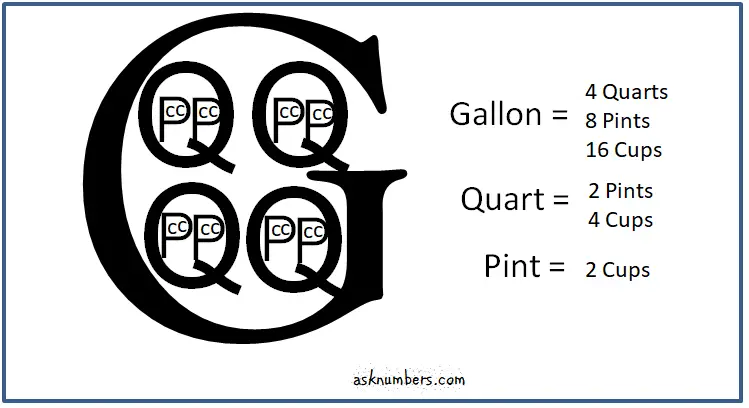# How Many Cups in A Quart, Pint or Gallon?

Are you wondering how many cups in a quart, pint or a gallon? Then you may find your answers here with a printable graphic to help you remember these common cooking unit conversions.

Gallons, quarts, pints and cups are units of liquid and dry measurement in United States Customary and the Imperial systems. Between these two measurement systems, the capacities of each of the units are different. The Imperial gallons, quarts or pints are larger than the US equivalents. For example, a US gallon is 3785.4 milliliters, whereas an Imperial gallon is 4546.09 milliliters. Similarly, a US quart is 946.35 mL and an Imperial quart is 1136.5 mL. A US pint is 473.17 mL and an Imperial pint is 568.26 mL.

But regardless of the measurement systems, there are 4 cups in a quart, 2 cups in a pint and 16 cups in a gallon. Just a note that, from early 1970's, the Imperial cups are replaced with the Metric cups but you may still find references to the Imperial cups in the old English recipe books.

Please find the printable graphic below to help you remember how many cups in a quart, in a pint or in a gallon.1 Gallon = 4 quarts, 8 pints, 16 cups

1 Quart = 2 pints, 4 cups

1 Pint = 2 cups

## HOW MANY CUPS IN A QUART?

There are 4 cups in a quart.

## HOW MANY CUPS IN A PINT?

There are 2 cups in a pint.

## HOW MANY CUPS IN A GALLON?

There are 16 cups in a gallon.

## HOW MANY QUARTS IN A GALLON?

There are 4 quarts in a gallon.

## HOW MANY PINTS IN A GALLON?

There are 8 pints in a gallon.

## HOW MANY PINTS IN A QUART?

There are 2 pints in a quart.

To convert between cups, quarts, pints and gallons, please visit volume units conversion.

Related Converters